FREE SHIPPING AUSTRALIA WIDE | PRICE MATCH GUARANTEE

# 2 Seater Dining Table

• Square - The average size of a square dining table for 2 is 76cm x 76cm• Round - The average size of a round dining table for 2 is between 76cmD - 92cmD• Rectangle/Oval - The average size of a rectangle/ oval dining table for 2 is 61cmW x 76cmL# 4 Seater Dining Table

• Square - The average size of a square dining table for 4 is 92cm x 92cm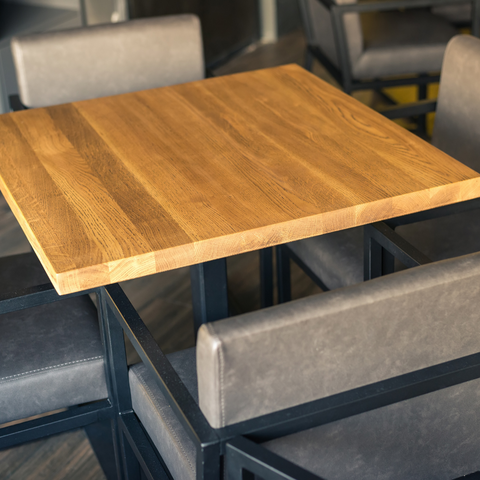• Round - The average size of a round dining table for 4 is between 92cmD - 122cmD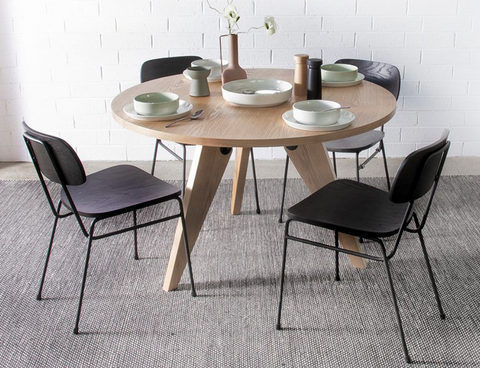• Rectangle/Oval - The average size of a rectangle/ oval dining table for 4 is 91cmW x 122cmL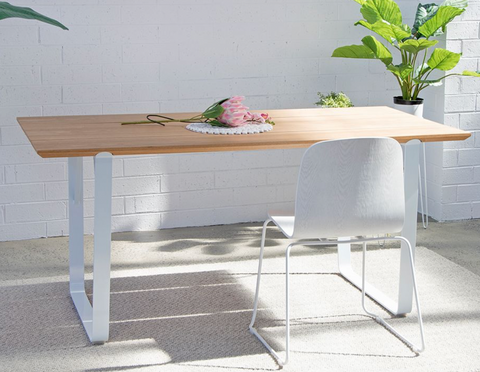# 6 Seater Dining Table

• Square - The average size of a square dining table for 6 is 122cm x 122cm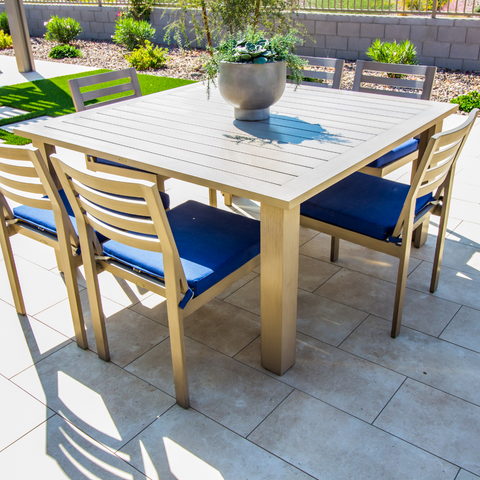• Round - The average size of a round dining table for 6 is between 137cmD - 152cmD• Rectangle/Oval - The average size of a rectangle/ oval dining table for 6 is 91cmW x 183cmL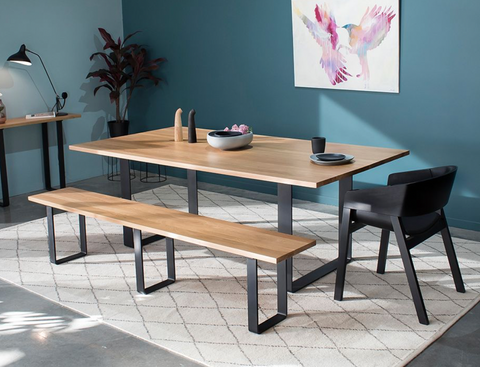Delux Dining 6 Seater Dining Table Range

# 8 Seater Dining Table

• Square - The average size of a square dining table for 8 is 183cm x 183cm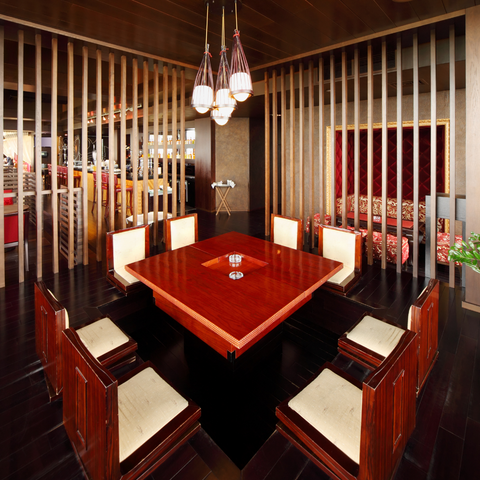• Round - The average size of a round dining table for 8 is between 152cmD - 183cmD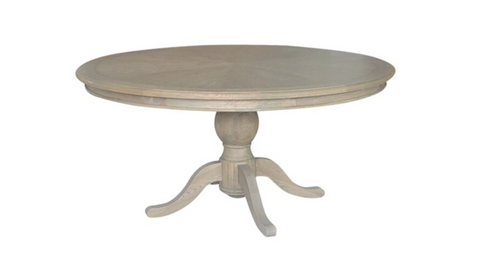• Rectangle/Oval - The average size of a rectangle/ oval dining table for 8 is 110cmW x 244cmLDelux Dining 8 Seater Dining Table Range

# 10 Seater Dining Table

• Square - The average size of a square dining table for 10 is 213cm x 213cm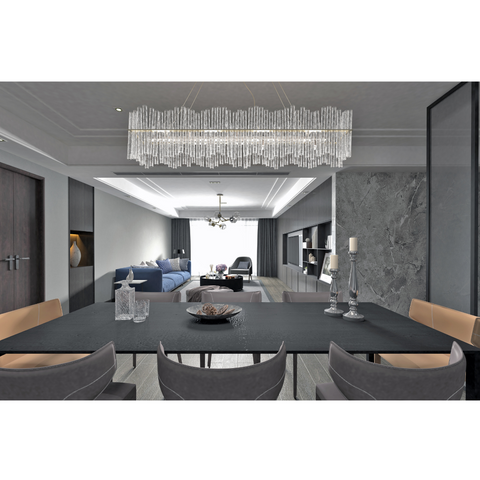• Round - The average size of a round dining table for 10 is between 213cmD - 244cmD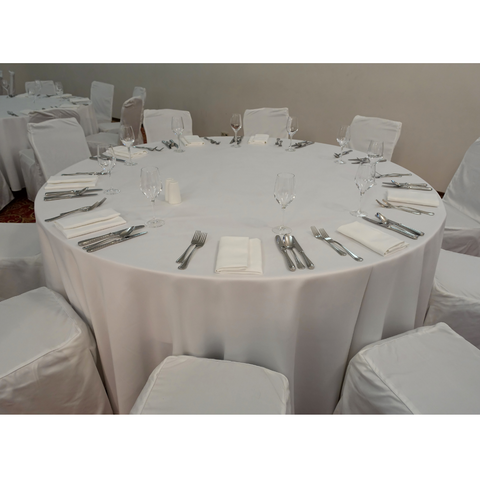• Rectangle/Oval - The average size of a rectangle/ oval dining table for 10 is 120cmW x 305cmL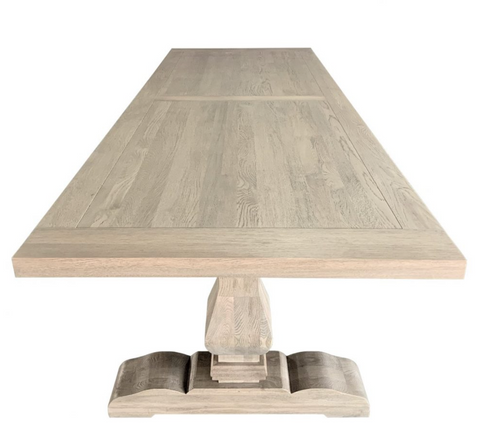# 12 Seater Dining Table

• Square - The average size of a square dining table for 12 is 244cm x 244cm
• Round - The average size of a round dining table for 12 is between 244cmD - 274cmD
• Rectangle/Oval - The average size of a rectangle/ oval dining table for 12 is 135cmW x 366cmL

Delux Dining 12 Seater Dining Table Range

Remember to take into consideration the size of your dining chairs, this will effect the amount of seats that can fit at a table. The sizing provided is to be used as a guide only.